### Waves and Light

In the previous quiz, we learned that waves are disturbances that transfer energy but not matter. Depending on the type, waves may or may not need a medium to travel and often take the form of periodic oscillations. In this quiz, we will examine the structure of waves that require a medium—mechanical waves. More specifically, we’ll learn about some of the different parts and properties of these waves.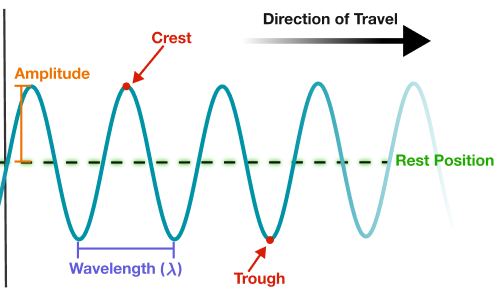A preview of some of the wave features we'll discuss

# Wave Anatomy

Mechanical waves are classified into different types depending on the direction of the disturbance. Let’s visualize this with the help of a Slinky. Like the rope from the last quiz, we can use the Slinky as a medium to see energy travel.

Suppose you’re holding the Slinky. You want to send energy down it, so you move your hand up and down and convert potential energy to kinetic energy. This energy travels down the Slinky forward. When you move your hand this way, the disturbance caused by the wave is up and down. In other words, the particles of the medium move perpendicular to the direction the wave travels in. The wave moves to the right, while the particles move up and down. This is an example of a transverse wave, a wave that displaces particles of the medium it travels in perpendicular (90°) to the direction of wave propagation.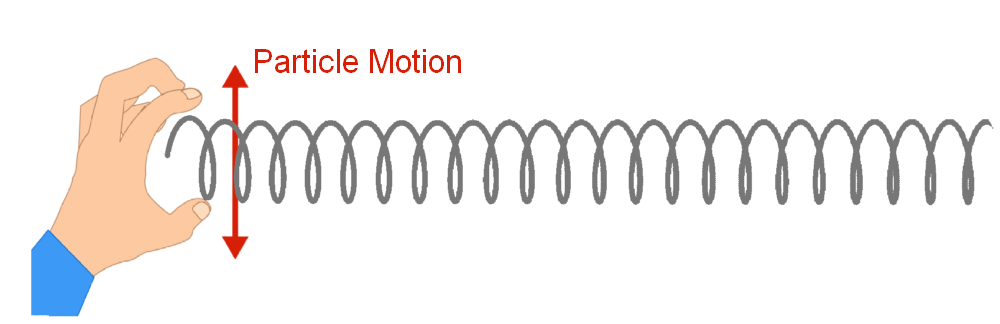# Wave Anatomy

But what happens if you move your hand left to right instead of up and down? Observe how the wave compares to the transverse wave we just saw. What can you conclude?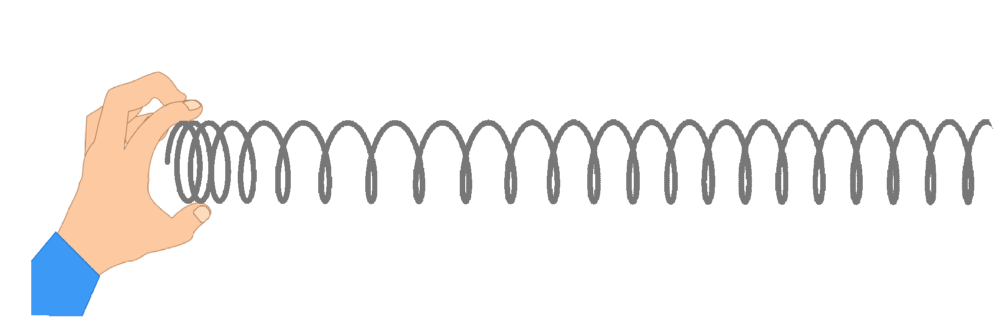# Wave Anatomy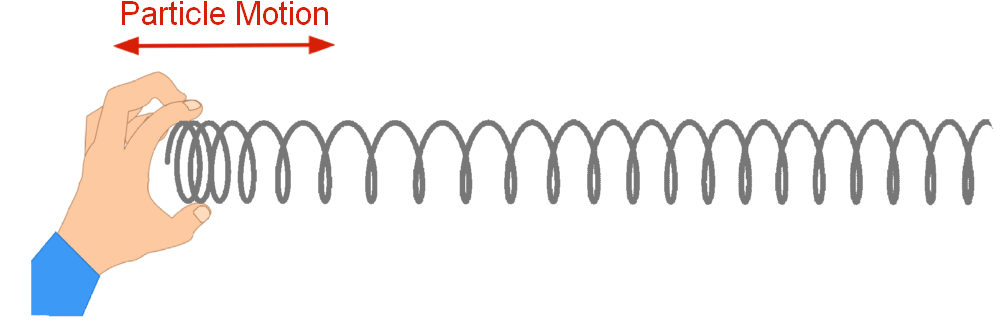A longitudinal wave A longitudinal wave is a wave that displaces particles of the medium it travels in parallel to the direction of wave propagation. Longitudinal waves travel in the same direction as the disturbance of particles.

Sound waves are longitudinal (when traveling through air and water). This means that the sounds we hear from speakers and the sounds emitted by whales are longitudinal! Remember that sound is the energy something releases when it vibrates. This energy travels through the air. For example, as a speaker vibrates, it transfers this energy to nearby air molecules. This causes the air molecules to move forward, pushing them closer together, and then backwards to their original (or resting) position. The vibrational sound energy continues to travel forward, disturbing new molecules of the medium. (However, each particle will not be transported with the energy; it will return to its original position. Particles are disturbed, not transported.) When the air particles are disturbed, it's in the same direction as (parallel to) the energy transferred.

We describe longitudinal waves as having compressions and rarefactions. A compression occurs when the wave compresses particles of the medium it travels in (pushes them close together). In our example, this is where the Slinky bunches together. On the other hand, a rarefaction is a region where the medium spreads out (particles separate). This is where the Slinky separates. These same compressions and rarefactions of particles occur when sound waves travel through a medium, such as air.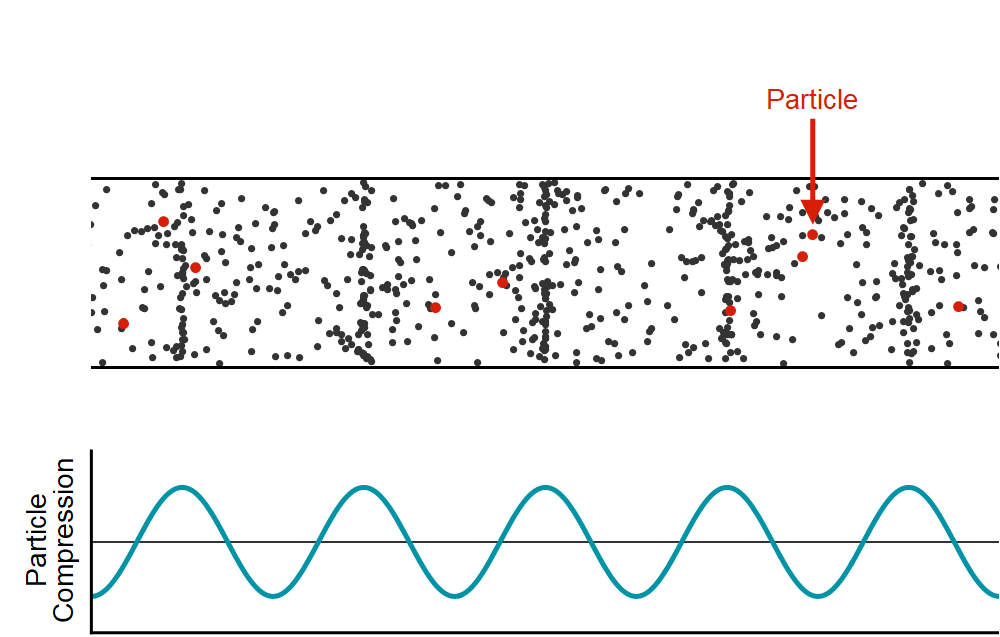Note: Although longitudinal waves displace particles by compression, we can still represent them with a periodically oscillating sine curve! However, in this case, the peaks (crests) indicate how much the particles are compressed. The crests are higher in the cases where the particles are compressed more.

# Wave Anatomy

Below are surface water waves. Not to be confused with the waves you see crashing on the beach, surface water waves occur out on the open ocean and are generated from wind that moves across the ocean’s surface. Watch the duck’s motion. Based on the duck’s motion, what can we conclude about the nature of surface water waves?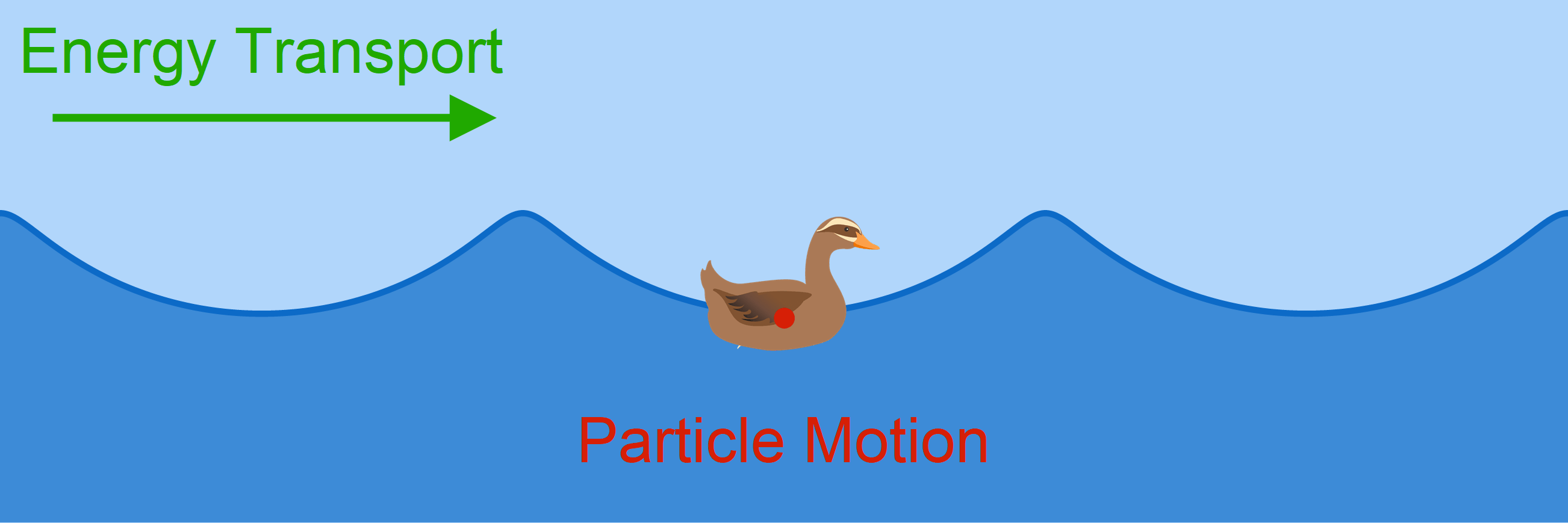# Wave Anatomy

Despite differences in particle displacement, all waves share a number of common properties. We’ll explore these characteristics so that we can better learn to describe waves.

Let’s start by learning about some basic wave anatomy using the labeled diagram below. The dotted line cutting across the wave represents the rest position of the wave (also referred to as the wave normal). It is the central position about which the wave oscillates. For a transverse wave on a rope, this is the height the rope assumes when it is at rest, and for a longitudinal wave, this is where the particles would be when they are neither compressed nor rarefied. In both cases, the rest position refers to the location of the particles before the wave arrives and the location they return to after.

The peaks of a wave are usually referred to as crests. More formally, a wave’s crests are the highest points (points of maximum upward displacement). On the other hand, the troughs of a wave are the lowest points (points of maximum downward displacement).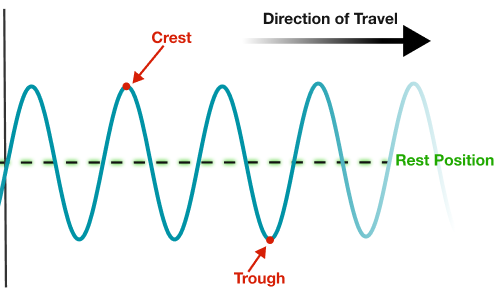# Wave Anatomy

The maximum displacement from the wave’s rest position is referred to as its amplitude $A.$ When a wave periodically oscillates, the amplitude is the displacement from the rest position to either a crest or a trough.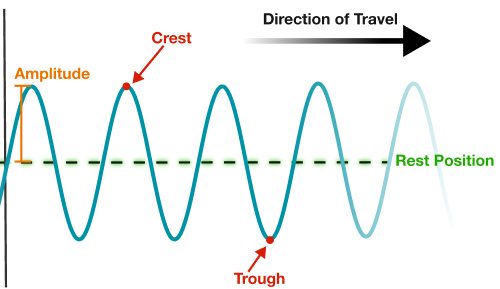In both transverse and longitudinal waves, the amplitude is based on particle displacement from the rest position. In a transverse wave, the amplitude is based on the particle displacement up or down from the rest position. In a longitudinal wave, the amplitude is based on the distance between particles where the wave compresses. The more compressed or rarefied the particles are as compared to their rest position, the greater the amplitude.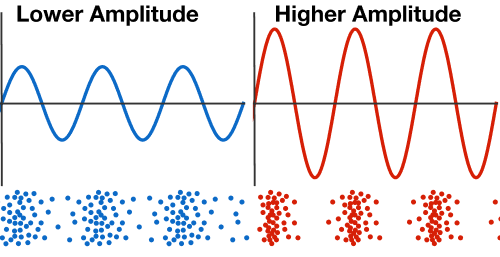# Wave Anatomy

Based on what you know about energy and your own intuition from everyday observation, how would you expect the energy of a mechanical wave to influence its amplitude?Hint: Consider which has more energy, a tsunami wave or a tiny pond ripple!

# Wave Anatomy

In mechanical waves, when energy increases, so does the amplitude. And this should make sense, as a higher energy wave will cause a greater disturbance of particles. Specifically, the energy of a mechanical wave is directly proportional to the square of the amplitude: $E \propto A^2.$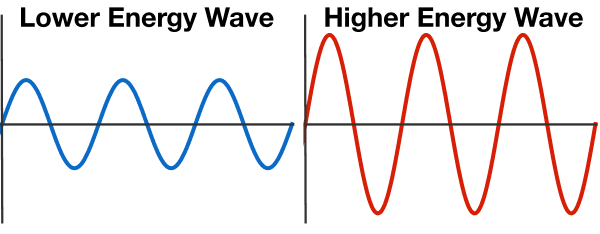Consider a wave in the water. When you think of a high energy wave, what does it look like? Is it big or small? A tsunami carries much more energy than a pond ripple. The amplitude of a tsunami is much greater than that of a pond ripple! In the images below, the large wave carries more energy than any of the pond ripples made by the bear! It's helpful to use our real-life experiences and intuition to inform our understanding of physics.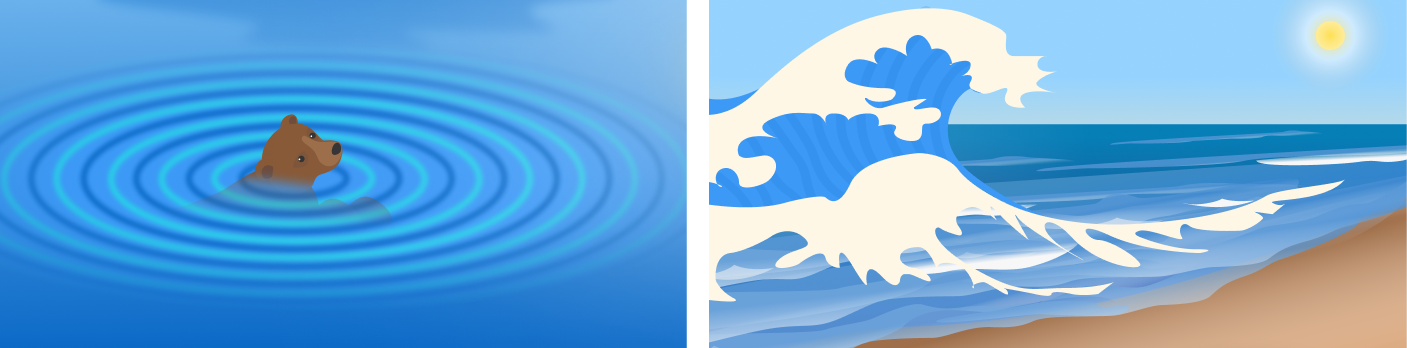# Wave Anatomy

Now let’s return to a characteristic of waves that we’re already familiar with—the wave’s period. We know that a period is the amount of time something takes to repeat. In the case of waves, the period $T$ actually refers to the length of time it takes to complete one wavelength, typically in seconds or milliseconds.

Wavelength $\lambda$ is the length of a complete wave cycle (the distance of one complete oscillation or pattern). This can be thought of as the distance between two neighboring crests or between any two corresponding points. While the wave’s period is a measure of time, wavelength is a measure of length. For example, we could measure a period in seconds using a stopwatch, but we measure wavelength as a length in meters, centimeters, nanometers, etc.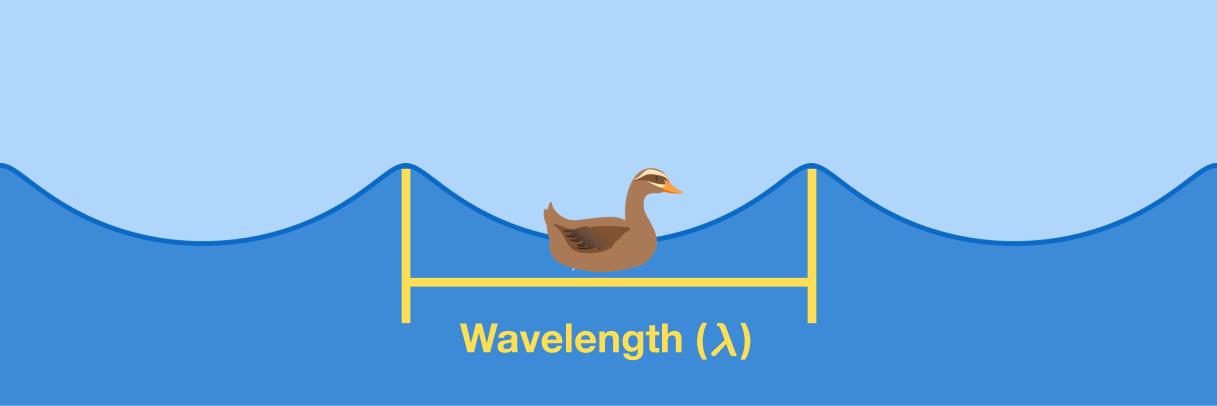# Wave Anatomy

Which of the following correctly orders the waves by wavelength from longest to shortest?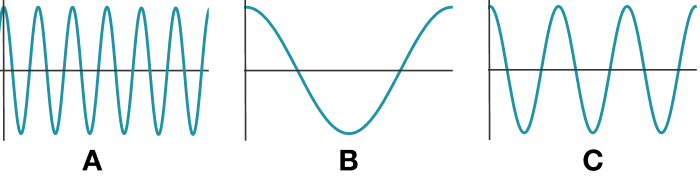# Wave Anatomy

In this quiz, we learned about basic wave anatomy. Some of the important takeaways include the following:

• Mechanical waves can be transverse, longitudinal, or a combination of both!
• A transverse wave is a wave that displaces particles of the medium it travels in perpendicular $(90^\circ)$ to the direction of wave propagation (travel).
• A longitudinal wave is a wave that displaces particles of the medium it travels in parallel to the direction of wave propagation by compressing particles.
• Amplitude $A$ is the maximum displacement from the wave’s rest position.
• Wave period $T$ is the time it takes to complete one wave cycle $($one wavelength $\lambda).$
• Wavelength $\lambda$ is the length of a complete wave cycle (the distance of one complete oscillation).

In the next quiz, we’ll apply some of what we’ve learned about basic wave anatomy to explore wave frequency and velocity.

# Wave Anatomy

×